# SOCR EduMaterials AnalysisActivities Wilcoxon

## SOCR Analysis - Two Independent Sample Wilcoxon-Mann-Whitney (U) Test

Given two groups of data, not necessarily with same sample size, we'd like to test the null hypothesis that the two groups are significantly different, statistically. This is a non-parametric test utilizing ranks of each datum, instead of the it's actual value.

### Two Independent Sample Wilcoxon Test Example

Go to the Wilcoxon-Mann-Whitney (U) Test Applet. We will demonstrate Two Independent Wilcoxon Test with a SOCR built-in example. This example is based on the data taken from "Mathematical Statistics and Data Analysis" by John A. Rice, Second Edition, Ducbury Press, 1995. (Page 390)

Note that this textbook uses the data for a Two Independent T-Test but we're only taking the data to demonstrate the Wilcoxon Two Independent Sample Rank Test.

Here's the steps of the activity:

1. Click on Two Independent Sample Wilcoxon Test at the left panel's combo box.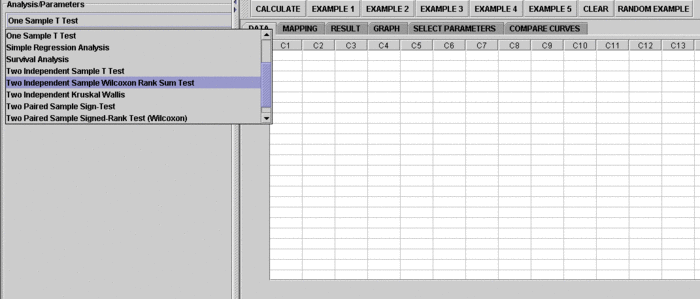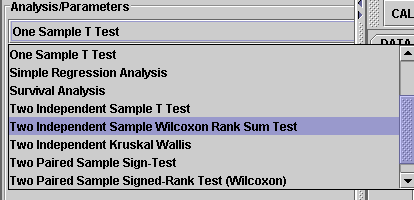On the right panel, first click on EXAMPLE 1 and next click on "Data" to retreive the demonstrated example. This is the example we'll be looking at.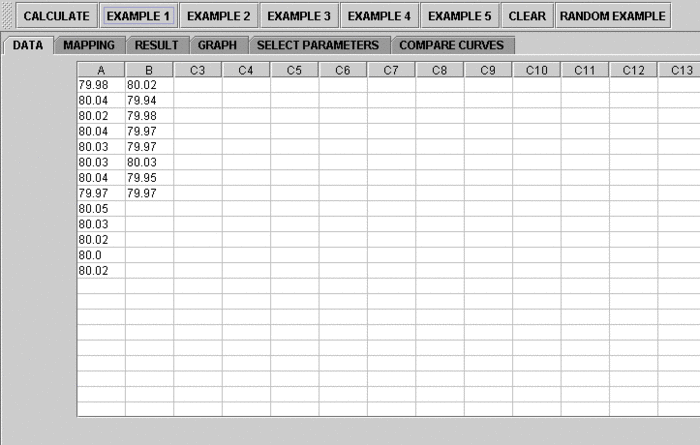2. Click on the "Mapping" button to get to the "Mapping" panel. Click on "ADD" under VARIABLE 1 to add A, and click on ADD under VARIABLE 2 to add B. We care about the difference, so which on goes to VARIABLE 1 or VARIABLE 2 is arbitrary. Here, the computer will set difference = Variable 2 - Variable 1. If you like the substraction to go the other way around, simple assign VARIABLE 1 and VARIABLE 2 in the opposite way.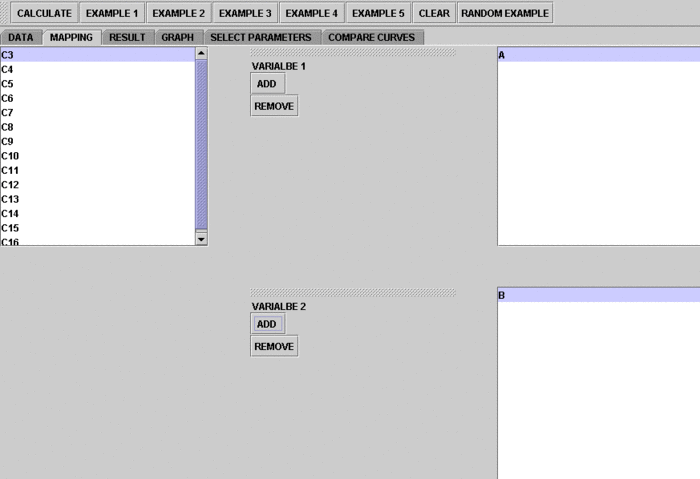3. Click on "Calculate" then "Result" to see the results.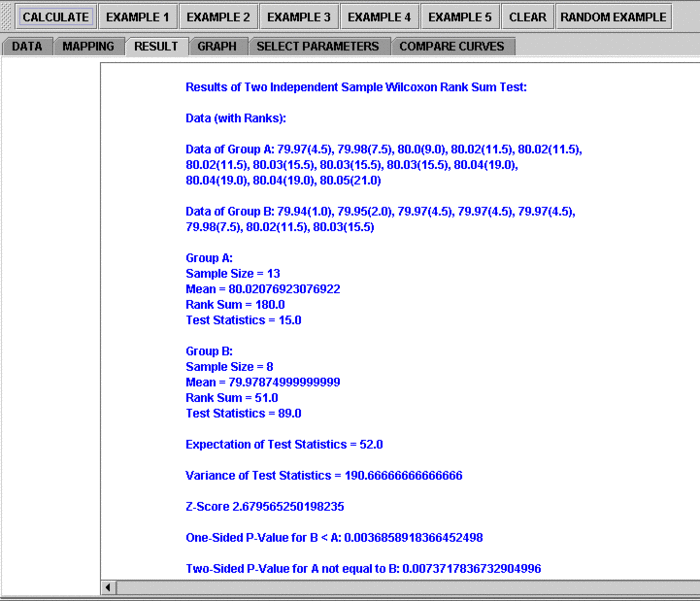Questions for students: By looking at the test results, what do you think about the data? What can you say about the two different shoe materials?